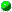Class com.oroinc.math.geometry.GeometricVector
`All Packages  Class Hierarchy  This Package  Previous  Next  Index`

# Class com.oroinc.math.geometry.GeometricVector

```java.lang.Object
|
+----com.oroinc.math.geometry.Tuple
|
+----com.oroinc.math.geometry.GeometricVector
```

public final class GeometricVector
extends Tuple
A class used to represent a geometric vector. It is named GeometricVector instead of Vector to avoid having to use explicit class names when also using java.util.Vector.

Copyright © 1996, 1997 Original Reusable Objects, Inc. All rights reserved.

Author:
Daniel F. Savarese

##GeometricVector()
Default constructor.GeometricVector(float, float)
Creates a two dimensional Vector.GeometricVector(float, float, float)
Creates a three dimensional Vector.GeometricVector(int)
Creates a GeometricVector of a given dimension and initizlies all of its coordinates to 0.GeometricVector(Tuple)
The GeometricVector copy constructor.

##assign(GeometricVector)
This is the assignment operator for GeometricVectors.clone()
Returns a deep copy of the GeometricVector.cross(Tuple, Tuple)
Calculate the cross product of two vectors and return the result.dot(Tuple, Tuple)
Calculate the dot product of two vectors and return the result.magnitude()
Returns the magnitude of the vector.normalize()
Normalizes the vector to a unit vector.normalized()
Creates a new vector in the same direction as the calling vector, but normalized to unit length.setDimension(int)
Sets the dimension of the GeometricVector.

##GeometricVector
```  public GeometricVector(Tuple tuple)
```
The GeometricVector copy constructor. Creates a new GeometricVector instance that is a deep copy of the argument. The programmer should exercise care in choosing what Tuple subclasses to pass as an argument.

Parameters:
tuple - The Tuple used to initialize the new GeometricVector.GeometricVector
```  public GeometricVector(int dimension)
```
Creates a GeometricVector of a given dimension and initizlies all of its coordinates to 0. Only dimensions 1 to 3 are supported and no check is made to ensure a valid argument was passed. It is the programmer's responsibility to use valid arguments.

Parameters:
dimension - The dimension of the new GeometricVector.GeometricVector
```  public GeometricVector(float x,
float y)
```
Creates a two dimensional Vector.

Parameters:
x - The x component.
y - The y component.GeometricVector
```  public GeometricVector(float x,
float y,
float z)
```
Creates a three dimensional Vector.

Parameters:
x - The x component.
y - The y component.
z - The z component.GeometricVector
```  public GeometricVector()
```
Default constructor. Creates a GeometricVector of dimension 0 under the assumption that a subsequent call to setDimension(int) will be made.

##cross
```  public final static GeometricVector cross(Tuple v1,
Tuple v2)
```
Calculate the cross product of two vectors and return the result. Assumes both vectors are 3 dimensional.

Parameters:
v1 - The first operand of the cross product.
v2 - The second operand of the cross product.
Returns:
The cross product of the two arguments.dot
```  public final static float dot(Tuple v1,
Tuple v2)
```
Calculate the dot product of two vectors and return the result.

Parameters:
v1 - The first operand of the dot product.
v2 - The second operand of the dot product.
Returns:
The dot product of the two arguments.assign
```  public void assign(GeometricVector vector)
```
This is the assignment operator for GeometricVectors. Assigns the value of the argument to the calling GeometricVector.

Parameters:
GeometricVector - The value to assign to the GeometricVector.clone
```  public Object clone()
```
Returns a deep copy of the GeometricVector. Essentially just returns
```new GeometricVector(this);
```

Returns:
A deep copy of the GeometricVector.
Overrides:
clone in class TuplesetDimension
```  public void setDimension(int dimension)
```
Sets the dimension of the GeometricVector. Only dimensions 1 to 3 are supported and no check is made to ensure a valid argument was passed. It is the programmer's responsibility to use valid arguments.

Parameters:
dimension - The new dimension of the GeometricVector.
Overrides:
setDimension in class Tuplemagnitude
```  public float magnitude()
```
Returns the magnitude of the vector. In ther words, the square root of its dot product.

Returns:
The magnitude of the vector.normalize
```  public void normalize()
```
Normalizes the vector to a unit vector. In other words, it multiplies the vector by the inverse of its magnitude.normalized
```  public GeometricVector normalized()
```
Creates a new vector in the same direction as the calling vector, but normalized to unit length.

Returns:
A normalized vector in the same direction as the caller.

`All Packages  Class Hierarchy  This Package  Previous  Next  Index`